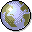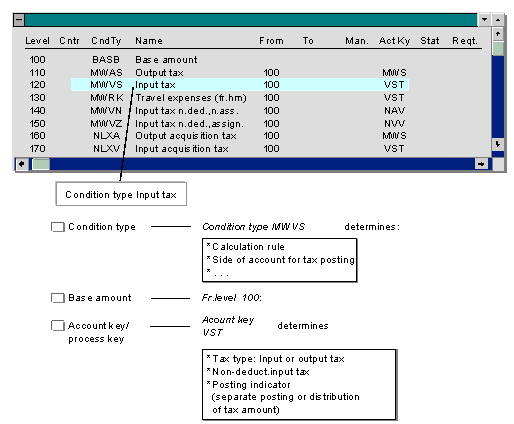Calculation Procedure: OverviewA calculation procedure is defined for each country, each containing the specifications required to calculate and post tax on sales/purchases. Each calculation procedure contains several tax types, which are called condition types in the procedure.

The system defaults condition types when you define a tax code. The condition type (such as input or output tax) specifies the base amount on which the tax is calculated and the account key that is used to post the tax. The specifications necessary for calculating and posting tax have been defined for the condition type and account key.The calculation procedure determines the amount on which the individual condition types are calculated. This can be the base amount (total of expense and revenue items) or a subtotal. Incoming acquisition tax, for example, is not calculated based on the expense or revenue items, but on the tax amount for outgoing acquisition tax. The column Fr.level determines the amount on which tax is calculated.